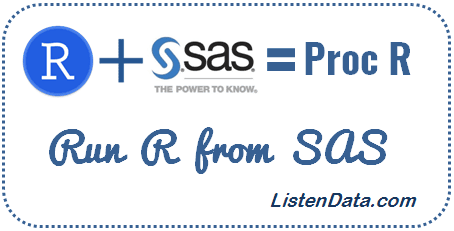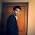# Run R from SAS

This tutorial explains how to call or run R code from SAS environment. Both SAS and R are leaders in statistical analysis tools for analytics industry. Both the tools have its own advantages and disadvantages. Life would be very easy if we can integrate R with SAS. SAS officially made it possible to run R code via PROC IML. But PROC IML is a paid SAS module which causes user to incur additional cost. R comes with a strong list of machine learning / text mining packages and advanced graphic capabilities which makes it stand apart from SAS. For example, you are asked to forecast 5 years sales. In SAS, you have to purchase SAS ETS module for forecasting procedures such as Proc ARIMA, Proc Forecast etc. In R, forecasting package is available for free and more powerful than SAS forecasting procedures.Run R from SAS
Xin Wei developed a macro called PROC_R which allows R language to be submitted with a SAS program in the base SAS environment. Check out the link for reference. It was designed similar to PROC SQL which lets users to write SQL queries on SAS datasets.

Steps to integrate R with SAS

Step 2 : Save the file in desired location.

Step 3 : Open the code and update the path of R executable file in the code below.
%macro quit(rpath=%str(C:\Progra~1\R\R-3.3.1\bin\R.exe))
Note : Make sure you use 'Progra~1' instead of 'Program Files' in the path. For example, my R executable file is saved in this path - C:\Program Files\R\R-3.3.1\bin\R.exe but i used this path - C:\Progra~1\R\R-3.3.1\bin\R.exe

Step 4 : Open Base SAS and call proc_R macro. Replace the existing path in the code with your own path where you have saved proc_R code.
%include "C:\Users\Deepanshu\Desktop\proc_R.sas";
Step 5 : Run R inside SAS environment. See the SAS program below -
%Proc_R(SAS2R=,R2SAS=);
cards4;
/********/
/*R Code*/
/********/
;;;;
%quit;

Proc_R Parameters

1. SAS2R   - specifies the names of SAS datasets to be converted to R dataframe. Can be single file name or  multiple files whose names are separated by space.
2. R2SAS   - specifies the names of R data frames to be converted to SAS datasets. Can be single file name or multiple files whose names are separated by space.

Examples : Proc_R

Create a sample data
data example;
input Srl x1-x5;
cards;
1 . 89 85 . 87
2 79 73 74 . .
3 80 82 . 85 .
;
run;
Example 1 : Calculating Summary Statistics

The following program first exports input data in CSV format and R code. Then it imports CSV data from the working directory into R and run the code you put inside %proc_R. Later it exports R log, data frame and image which are later imported in SAS. Here, we are telling SAS to integrate with R to calculate summary statistics on example dataset.
%include "C:\Users\Deepanshu\Desktop\proc_R.sas";

/*Run Summary Statistics*/
%Proc_R(SAS2R=example,R2SAS=);
cards4;
setwd("C:/Users/Deepanshu/Documents")
summary(example)
;;;;
%quit;

proc print data=rlog;
run;
Note : It is required to set the working directory with setwd() function. Update your directory in the setwd() function.Summary displayed in SAS result window

The output shown in the image above is a truncated one as the real output is large in size.

Example 2 : Data Manipulation with dplyr Package

In the program below, we are loading dplyr library and calculating first non-missing record in each row. The calculation would be done in R and later SAS exports dataframe df.
%Proc_R(SAS2R=example,R2SAS=df);
cards4;
setwd("C:/Users/Deepanshu/Documents")
library(dplyr)
df = mutate(example,nonmiss=coalesce(x1,x2,x3,x4,x5))
;;;;
%quit;

Example3 : Creating Bar Chart

The following code creates a bar chart in R and later displayed in SAS Results Viewer.
%Proc_R(SAS2R=,R2SAS=);
cards4;
setwd("C:/Users/Deepanshu/Documents")
mydata = sample(LETTERS[1:5],16,replace = TRUE)
mydata.count= table(mydata)
barplot(mydata.count)
colors = c("red", "yellow", "green", "violet", "orange", "blue", "pink", "cyan")
barplot(mydata.count, col=colors, main="Main Title ", xlab="X-Axis Title")
;;;;
%quit;

Example 4 : Build ARIMA Model in R
data arimaexample;
input sales @@;
cards;
360 358 357
374 371 385
385 389 398
400 412 424
418 412 408
420 424 438
435 438 446
451 456 470
457 448 440
456 457 469
473 477 483
490 498 503
;
run;
%Proc_R(SAS2R=arimaexample,R2SAS=Forecastmodel);
cards4;
setwd("C:/Users/Deepanshu/Documents")
library(forecast)

# Convert it to ts object
dat = data.frame(arimaexample)
tsdata = ts(dat)

# Plot time series data
plot.ts(tsdata)
tsdisplay(tsdata)

# Box lambda Transformation
lambda = BoxCox.lambda(tsdata)

#lambda close to 1 means no transformation required
tsdata2 = BoxCox(tsdata, lambda=lambda)
plot.ts(tsdata2)

# Unit Ratio Tests
library(tseries)
kpss = kpss.test(tsdata2)

# Number of Difference Required to make data stationary
ndiffs(tsdata2)
tsdata3 = diff(tsdata2, differences = 1)
final = auto.arima(tsdata, trace= TRUE, ic ="bic", approximation = FALSE, stepwise = FALSE)

# predict the next 5 periods
Forecastmodel = forecast.Arima(final, h = 5)

;;;;
%quit;

proc print data= rlog;
run;

Example 5 : Supports Multiple datasets / dataframes

In the macro, we can also specify multiple SAS datasets or data frames. In the code below, we have specified multiple SAS datasets that would be imported into R and then perform some calculation and later multiple R data frames would be exported.
%Proc_R(SAS2R=example arimaexample,R2SAS= df1 df2);
cards4;
setwd("C:/Users/Deepanshu/Documents")
df1=data.frame(mean(as.numeric(arimaexample\$sales)))
df2=data.frame(sum(is.na(example)))
;;;;
%quit;
proc print data = df1;
proc print data = df2;
run;Related PostsDeepanshu founded ListenData with a simple objective - Make analytics easy to understand and follow. He has over 8 years of experience in data science. During his tenure, he has worked with global clients in various domains like Banking, Insurance, Telecom and Human Resource.

12 Responses to "Run R from SAS"
1.Very interesting post. I have other examples of integrating SAS and R
in my SAS blog (in spanish):
sasybi.blogspot.com

2.should R be needed to be installed prior to this change?

1.Yes R is required before submitting the above code.

3.Could R be integrated with SAS VA tool?

1.No I don't think so.

4.Hi,
I'm running Proc_R on windows 7, SAS 9.4 TS1M3 and R-3.1.2 x64, and the following error appeared:

WARNING: Apparent symbolic reference FGNAME not resolved.
fgname
WARNING: Apparent symbolic reference FGSW not resolved.
ERROR: A character operand was found in the% EVAL function or% IF condition where numeric operand is required. The condition was:
& fgsw = 1
ERROR: The macro QUIT will stop executing.

Can you help me?

Thank you.

Tnylson

1.Hi I found the error, had typed C:\Progra~1\R\R-3.1.2\bin\R.exe instead of C:\Progra~1\R\R-3.1.2\bin\x64\R.exe

Thank you

Tnylson

2.Glad it worked for you. Cheers!

5.What if R isn't installed under program files? Will this still work?

For example, my work computer has R under C:/Users/myname...

I can't change this. What exactly does Progra~1 do?

1.Answered my own question. You can run from another directory that isn't program files. And Progra~1 seems to be a shorthand way of referencing program files.

6.Your website is very good and nice information was provided in your site, thanks for sharing.
R Programming Training in Ameerpet, Hyderabad

7.Hi, i ran this code but i got error like

ERROR: No DATALINES or INFILE statement.
ERROR: The _INFILE_ variable has been referenced, but no DATALINES or INFILE statement was
found.

ERROR: Physical file does not exist, C:\Users\SM-B-L~1.DEL\AppData\Local\Temp\SAS Temporary
Files\_TD8300_SM-B-LP-015-SAR_\r_code1849969176.r.
ERROR: A component of C:\Users\SM-B-L~1.DEL\AppData\Local\Temp\SAS Temporary
Files\_TD8300_SM-B-LP-015-SAR_\C:\Users\SM-B-L~1.DEL\AppData\Local\Temp\SAS Temporary
Files\_TD8300_SM-B-LP-015-SAR_\rhtml_1849969177.html is not a directory.
ERROR: No body file. HTML output will not be created.
this thing .

can you help me out those kind of problem.

Next → ← Prev
Love this Post? Spread the Word!
Share